# T Function

Tests if the value provided is a text or not

## What is the T Function?

The T function is categorized under Excel Text functions. It will test if the value provided is text or not. If it is text, then the function will return the text. Otherwise, it will return an empty text string. T is one of two functions in Excel that contains only one character.

As a financial analyst, this function is not as useful as many other functions, as Microsoft Excel automatically converts values as necessary. It is provided for compatibility with other spreadsheet programs.

### Formula

=T(value)

The T function uses only one argument:

1. Value (required argument) – This is the value we wish to test.

### How to use the T Function in Excel?

To understand the uses of the function, consider an example:

#### Example 1

Suppose we are given the following data: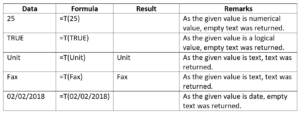If the cell contains anything other than text, the T function will return an empty string. Hence, we got empty cells for numbers, dates, and logical value.

We get the results below: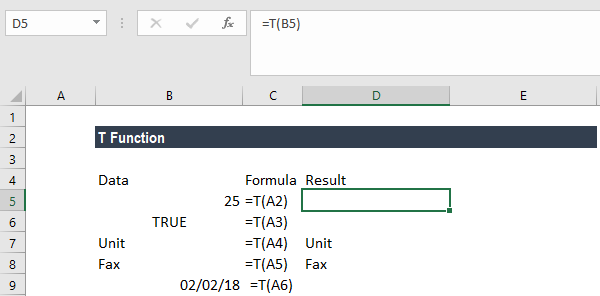#### Example 2

Suppose we are given the data below: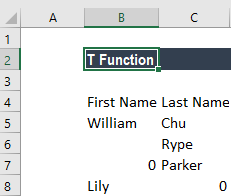If we wish to combine the first name and last name, we should use the CONCATENATE function. However, there are zero’s in rows 4 and 5. In this scenario, the T function can be used. The formula used is shown below: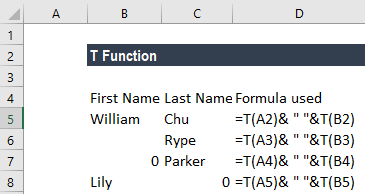We get the results below: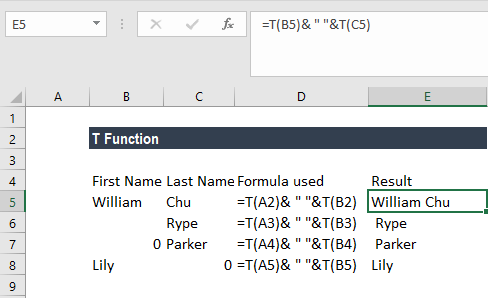If we use the CONCATENATE function in row 7, the result would be “0 Parker.” This would be untidy and of no practical use. Therefore, we used the T function.

Thanks for reading CFI’s guide to important Excel functions! By taking the time to learn and master these functions, you’ll significantly speed up your financial analysis. To learn more, check out these additional CFI resources:

• Excel Functions for Finance